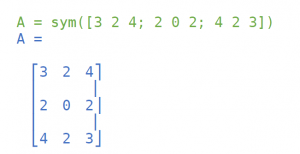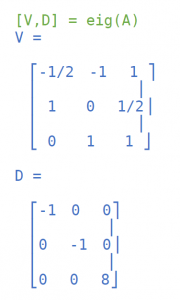# Example of Eigenvalues and Eigenvectors MATLAB

We hope that our sample for eigenvalues and eigenvectors MATLAB will help you with your own homework. However, if you have absolutely no time, effort, or desire, then tasks of this kind can’t be mastered even formally. In this case, AssignmentShark.com offers “do my math homework for me free” help. Consider our service as a helper, because we are here to help you become successful in your study. On our site, you can choose an expert on your own, or we can find the most suitable one for your assignment.

We have a large team of experts knowledgeable in different spheres of study. Ordering is not difficult – place your order with the requirements and the deadline and get your assignment done in the shortest possible time. We can help with assignments you if you have difficult moments in your study. We are available 24/7, so you can contact us any time you want. Read the sample below to find out how our experts complete such assignments.

Find the eigenvectors and eigenvalues of the following matrix in MATLAB:

Solution:

MATLAB can compute eigenvalues and eigenvectors of a square matrix, either numerically or symbolically.

Numerical eigenvalues and eigenvectors

(Note: green color marks user input, and blue color is MATLAB response.)

First let’s set matrix:

A = [3 2 4; 2 0 2; 4 2 3]

A =

 3 2 4 2 0 2 4 2 3

The “eig” command computes the eigenvalues and eigenvectors:

[V,D] = eig(A)

 V = -0.49410 -0.55805 0.66667 -0.47202 0.81614 0.33333 0.73011 0.14998 0.66667 D = Diagonal Matrix -1.00000 0 0 0 -1.00000 0 0 0 8.00000

The “eig” command returns two matrices. The first contains the eigenvectors as the columns of the matrix, while the second is a diagonal matrix with the eigenvalues on the diagonal. The eigenvectors and eigenvalues are given in the same order.

We can also call the “eig” command with a single output, in which case only the eigenvalues are returned, and in a vector instead of a matrix:

ev = eig(A)

ev =

-1.00000

-1.00000

8.00000

Symbolical eigenvalues and eigenvectors

To obtain symbolic (exact) eigenvalues and eigenvectors, it is only necessary to define the matrix to be symbolic:The computation then proceeds exactly as before: